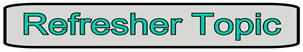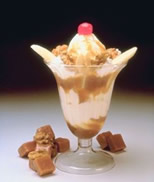Counting Principle MathBitsNotebook.com Terms of Use   Contact Person: Donna RobertsWhen dealing with the occurrence of more than one event (a compound event), it is important to be able to quickly determine how many possible outcomes exist.
What will be the size of the sample space?For example, if ice cream sundaes come in 5 flavors with 4 possible toppings, how many different sundaes can be made with one flavor of ice cream and one topping?

 In an attempt to find the "count" of how many sundaes are possible, a tree diagram could be drawn or a list of all possible sample space entries could be created. Unfortunately, these processes can be very time consuming when dealing with a large number of options. There is a short cut method! To count the combinations of ice cream and toppings, simply multiply the number of options for each event:    5 • 4 = 20 possible sundaes. This simple multiplication process is known as the Counting Principle.The Fundamental Counting Principle:  If there are a ways for one activity to occur, and b ways for a second activity to occur, then there are a • b ways for both to occur.

NOTE: The Counting Principle only works when all of the choices are independent of each other.
One choice does not depend upon another choice.
If one choice affects another choice, then this simple multiplication process will not yield the correct answer.

 Examples: 1.  Activities:  roll a die and flip a coin      There are 6 ways to roll a die and 2 ways to flip a coin.      There are 6 • 2 = 12 ways to roll a die and flip a coin.      (The sample space will contain a count of 12 possible outcomes.) 2.  Activities:  draw two cards from a standard deck of 52 cards without replacing the cards      There are 52 ways to draw the first card.      There are 51 ways to draw the second card.      There are 52 • 51 = 2,652 ways to draw the two cards.      (The sample space will contain a count of 2,652 possible outcomes.) The Counting Principle also works for more than two activities.   3.  Activities:  a coin is tossed five times      There are 2 ways to flip each coin.      There are 2 • 2 • 2 • 2  •2 = 32 arrangements of heads and tails.      (The sample space will contain a count of 32 possible outcomes.) 4.  Activities: a die is rolled four times      There are 6 ways to roll each die.      There are 6 • 6 • 6 • 6 = 1,296 possible outcomes.      (The sample space will contain a count of 1,296 possible outcomes.) So, when does the Counting Principle NOT work? 5.  Activities: You are buying a new skateboard.      There are 2 styles: wood or polymer.      There are 4 colors: red, black, blue, orange.      There are 3 designs: line art, tattoo, splash      There are 2 • 4 • 3 = 24 possible choices of skateboards. Yes, find and dandy!!  BUT... the salesman says he is sorry but they are all sold out of all orange polymer skateboards. Now, how many choices do you have? Your choices are no longer independent of each other. The color options now depend upon which style board is chosen, wood or polymer. Well, you still have all of the wood skateboard options which will be 1 • 4 • 3 = 12 choices. Under the polymer skateboards, you now have 1• 3 • 3 = 9 choices. This gives a total of 21 choices.

 The Counting Principle is easy! Simply MULTIPLY the number of ways each event can occur to find the sample space count. (events are independent)